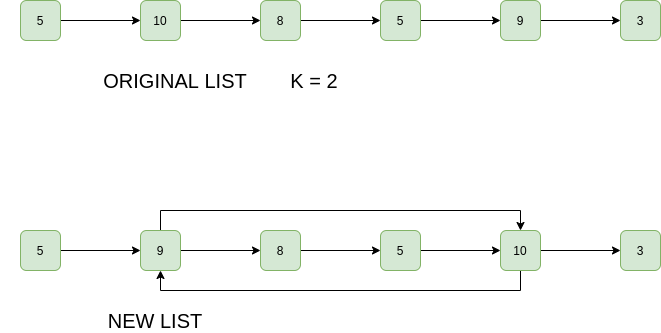Open in App
Not now

# Python Program For Swapping Kth Node From Beginning With Kth Node From End In A Linked List

• Last Updated : 19 May, 2022

Given a singly linked list, swap kth node from beginning with kth node from end. Swapping of data is not allowed, only pointers should be changed. This requirement may be logical in many situations where the linked list data part is huge (For example student details line Name, RollNo, Address, ..etc). The pointers are always fixed (4 bytes for most of the compilers).Example:

```Input: 1 -> 2 -> 3 -> 4 -> 5, K = 2
Output: 1 -> 4 -> 3 -> 2 -> 5
Explanation: The 2nd node from 1st is 2 and
2nd node from last is 4, so swap them.

Input: 1 -> 2 -> 3 -> 4 -> 5, K = 5
Output: 5 -> 2 -> 3 -> 4 -> 1
Explanation: The 5th node from 1st is 5 and
5th node from last is 1, so swap them.```

Illustration:Approach: The idea is very simple find the k th node from the start and the kth node from last is n-k+1 th node from start. Swap both the nodes.
However there are some corner cases, which must be handled

1. Y is next to X
2. X is next to Y
3. X and Y are same
4. X and Y don’t exist (k is more than number of nodes in linked list)

Below is the implementation of the above approach.

## Python3

 `""" ` `A Python3 program to swap kth node from  ` `the beginning with kth node from the end ` `"""` `class` `Node: ` `    ``def` `__init__(``self``, data,  ` `                 ``next` `=` `None``): ` `        ``self``.data ``=` `data ` `        ``self``.``next` `=` `next` `     `  `class` `LinkedList: ` `    ``def` `__init__(``self``, ``*``args, ``*``*``kwargs): ` `        ``self``.head ``=` `Node(``None``) ` ` `  `    ``""" ` `    ``Utility function to insert a node at  ` `    ``the beginning ` `    ``@args: ` `        ``data: value of node ` `    ``"""` `    ``def` `push(``self``, data): ` `        ``node ``=` `Node(data) ` `        ``node.``next` `=` `self``.head ` `        ``self``.head ``=` `node ` `     `  `    ``# Print linked list ` `    ``def` `printList(``self``): ` `        ``node ``=` `self``.head ` ` `  `        ``while` `node.``next` `is` `not` `None``: ` `            ``print``(node.data, end ``=` `" "``) ` `            ``node ``=` `node.``next` `     `  `    ``# count number of node in linked list ` `    ``def` `countNodes(``self``): ` `        ``count ``=` `0` `        ``node ``=` `self``.head ` `        ``while` `node.``next` `is` `not` `None``: ` `            ``count ``+``=` `1` `            ``node ``=` `node.``next` `        ``return` `count ` `     `  `    ``""" ` `    ``Function for swapping kth nodes from ` `    ``both ends of linked list ` `    ``"""` `    ``def` `swapKth(``self``, k): ` ` `  `        ``# Count nodes in linked list ` `        ``n ``=` `self``.countNodes() ` ` `  `        ``# Check if k is valid ` `        ``if` `nnext  ` `        ``is x, in this case, x_prev and y are same.  ` `        ``So the statement "x_prev->next = y" creates  ` `        ``a self loop. This self loop will be broken ` `        ``when we change y->next.  ` `        ``"""` `        ``if` `x_prev ``is` `not` `None``: ` `            ``x_prev.``next` `=` `y ` ` `  `        ``# Same thing applies to y_prev ` `        ``if` `y_prev ``is` `not` `None``: ` `            ``y_prev.``next` `=` `x ` `         `  `        ``""" ` `        ``Swap next pointers of x and y. These  ` `        ``statements also break self loop if  ` `        ``x->next is y or y->next is x  ` `        ``"""` `        ``temp ``=` `x.``next` `        ``x.``next` `=` `y.``next` `        ``y.``next` `=` `temp ` ` `  `        ``# Change head pointers when k is 1 or n ` `        ``if` `k ``=``=` `1``: ` `            ``self``.head ``=` `y ` `         `  `        ``if` `k ``=``=` `n: ` `            ``self``.head ``=` `x ` ` `  `# Driver Code ` `llist ``=` `LinkedList() ` `for` `i ``in` `range``(``8``, ``0``, ``-``1``): ` `    ``llist.push(i) ` `llist.printList() ` ` `  ` `  `for` `i ``in` `range``(``1``, ``9``): ` `    ``llist.swapKth(i) ` `    ``print``(``"Modified List for k = "``, i) ` `    ``llist.printList() ` `    ``print``("") ` `# This code is contributed by Pulkit `

Output:

```Original Linked List: 1 2 3 4 5 6 7 8

Modified List for k = 1
8 2 3 4 5 6 7 1

Modified List for k = 2
8 7 3 4 5 6 2 1

Modified List for k = 3
8 7 6 4 5 3 2 1

Modified List for k = 4
8 7 6 5 4 3 2 1

Modified List for k = 5
8 7 6 4 5 3 2 1

Modified List for k = 6
8 7 3 4 5 6 2 1

Modified List for k = 7
8 2 3 4 5 6 7 1

Modified List for k = 8
1 2 3 4 5 6 7 8```

Complexity Analysis:

• Time Complexity: O(n), where n is the length of the list.
One traversal of the list is needed.
• Auxiliary Space: O(1).
No extra space is required.

Please refer complete article on Swap Kth node from beginning with Kth node from end in a Linked List for more details!

My Personal Notes arrow_drop_up
Related Articles